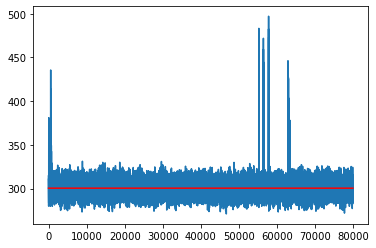# Advanced TPS Analysis on Alanine Dipeptide

The following example demonstrates some more advanced analysis that can be performed with OpenPathSampling. This example assumes that you have already run both the flexible length TPS example on alanine dipeptide and the fixed length TPS example on alanine dipeptide.

Now we'll move on to a few more advanced analysis techniques. (These are discussed in Paper II.)

With the fixed path length ensemble, we should check for recrossings. To do this, we create an ensemble which represents the recrossing paths: a frame in $\beta$, possible frames in neither $\alpha$ nor $\beta$, and then a frame in $\alpha$.

Then we check whether any subtrajectory of a trial trajectory matches that ensemble, by using the Ensemble.split() function. We can then further refine to see which steps that included trials with recrossings were actually accepted.

In :
from __future__ import print_function
%matplotlib inline
import openpathsampling as paths
import numpy as np
import matplotlib.pyplot as plt
from tqdm.auto import tqdm
import os
import openpathsampling.visualize as ops_vis
from IPython.display import SVG

In :
%%time

CPU times: user 8.36 s, sys: 1.61 s, total: 9.97 s
Wall time: 34.6 s

In :
%%time

CPU times: user 24.6 s, sys: 5.35 s, total: 30 s
Wall time: 1min 40s

In :
flex_network = flexible.networks['tps_network']

In :
# create the ensemble that identifies recrossings
alpha = fixed.volumes['C_7eq']
beta = fixed.volumes['alpha_R']
recrossing_ensemble = paths.SequentialEnsemble([
paths.LengthEnsemble(1) & paths.AllInXEnsemble(beta),
paths.OptionalEnsemble(paths.AllOutXEnsemble(alpha | beta)),
paths.LengthEnsemble(1) & paths.AllInXEnsemble(alpha)
])

In :
%%time
# now we check each step to see if its trial has a recrossing
steps_with_recrossing = []
for step in tqdm(fixed.steps):
# trials is a list of samples: with shooting, only one in the list
recrossings = [] # default for initial empty move (no trials in step.change)
for trial in step.change.trials:
recrossings = recrossing_ensemble.split(trial.trajectory)
# recrossing contains a list with the recrossing trajectories
# (len(recrossing) == 0 if no recrossings)
if len(recrossings) > 0:
steps_with_recrossing += [step]  # save for later analysis

CPU times: user 27min 18s, sys: 7.71 s, total: 27min 26s
Wall time: 27min 54s

In :
accepted_recrossings = [step for step in steps_with_recrossing if step.change.accepted is True]

In :
print("Trials with recrossings:", len(steps_with_recrossing))
print("Accepted trials with recrossings:", len(accepted_recrossings))

Trials with recrossings: 532
Accepted trials with recrossings: 113


Note that the accepted trials with recrossing does not account for how long the trial remained active. It also doesn't tell us whether the trial represented a new recrossing event, or was correlated with the previous recrossing event.

Let's take a look at one of the accepted trajectories with a recrossing event. We'll plot the value of $\psi$, since this is what distinguishes the two states. We'll also select the frames that are actually inside each state and color them (red for $\alpha$, blue for $\beta$).

In :
psi = fixed.cvs['psi']
trajectory = accepted_recrossings.active.trajectory
in_alpha_indices = [trajectory.index(s) for s in trajectory if alpha(s)]
in_alpha_psi = [psi(trajectory)[i] for i in in_alpha_indices]
in_beta_indices = [trajectory.index(s) for s in trajectory if beta(s)]
in_beta_psi = [psi(trajectory)[i] for i in in_beta_indices]

plt.plot(psi(trajectory), 'k-')
plt.plot(in_alpha_indices, in_alpha_psi, 'ro')  # alpha in red
plt.plot(in_beta_indices, in_beta_psi, 'bo')  # beta in blue

Out:
[<matplotlib.lines.Line2D at 0x14a421da4d10>]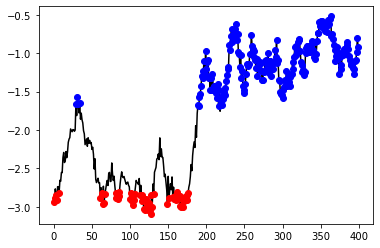Now let's see how many recrossing events there are in each accepted trial. If there's one recrossing, then the trajectory must go $\alpha\to\beta\to\alpha\to\beta$ to be accepted. Two recrossings would mean $\alpha\to\beta\to\alpha\to\beta\to\alpha\to\beta$.

In :
recrossings_per = []
for step in accepted_recrossings:
for test in step.change.trials:
recrossings_per.append(len(recrossing_ensemble.split(test.trajectory)))

In :
print(recrossings_per)

[1, 1, 1, 1, 1, 1, 1, 1, 1, 1, 1, 1, 1, 1, 1, 1, 1, 1, 1, 1, 1, 1, 1, 1, 1, 1, 1, 1, 1, 1, 1, 1, 1, 1, 1, 1, 1, 1, 1, 1, 1, 1, 1, 1, 1, 1, 1, 1, 1, 1, 1, 1, 1, 1, 1, 1, 1, 1, 1, 1, 1, 1, 1, 1, 1, 1, 1, 1, 1, 1, 1, 1, 1, 1, 1, 1, 1, 1, 1, 1, 1, 1, 1, 1, 1, 1, 1, 1, 1, 1, 1, 1, 1, 1, 1, 1, 1, 1, 1, 1, 1, 1, 1, 1, 1, 1, 1, 1, 1, 1, 1, 1, 1]

In :
# these numbers come from accepted trial steps, not all steps
print(sum(recrossings_per))
print(len(recrossings_per))
print(len([x for x in recrossings_per if x==2]))

113
113
0


# Comparing the fixed and flexible simulations¶

In :
%%time
# transition path length distribution
flex_ens = flex_network.sampling_ensembles
fixed_transition_segments = sum([flex_ens.split(step.active.trajectory)
for step in tqdm(fixed.steps)],[])
fixed_transition_length = [len(traj) for traj in fixed_transition_segments]

CPU times: user 6h 11min 58s, sys: 1min 21s, total: 6h 13min 20s
Wall time: 6h 16min 54s

In :
flexible_transition_length = [len(s.active.trajectory) for s in flexible.steps]

In :
print(len(fixed_transition_length))

10232

In :
bins = np.linspace(0, 400, 80);
plt.hist(flexible_transition_length, bins, alpha=0.5, density=True, label="flexible");
plt.hist(fixed_transition_length, bins, alpha=0.5, density=True, label="fixed");
plt.legend(loc='upper right');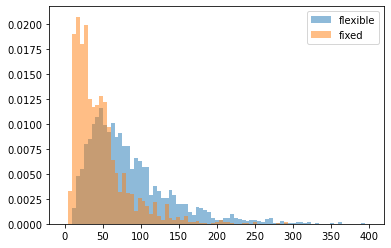#### Identifying different mechanisms using custom ensembles¶

We expected the plot above to be very similar for both cases. However, we know that the $\alpha\to\beta$ transition in alanine dipeptide can occur via two mechanisms: since $\psi$ is periodic, the transition can occur due to an overall increase in $\psi$, or due to an overall decrease in $\psi$. We also know that the alanine dipeptide transitions aren't actually all that rare, so they will occur spontaneously in long simulations.

This section shows how to create custom ensembles to identify whether the transition occurred with an increasing $\psi$ or a decreasing $\psi$. We also need to account for (unlikely) edge cases where the path starts in one direction but completes the transition from the other.

First, we'll create a few more Volume objects. In this case, we will completely tile the Ramachandran space; while a complete tiling isn't necessary, it is often useful.

In :
# first, we fully subdivide the Ramachandran space
phi = fixed.cvs['phi']
deg = 180.0/np.pi
nml_plus = paths.PeriodicCVDefinedVolume(psi, -160/deg, -100/deg, -np.pi, np.pi)
nml_minus = paths.PeriodicCVDefinedVolume(psi, 0/deg, 100/deg, -np.pi, np.pi)
nml_alpha = (paths.PeriodicCVDefinedVolume(phi, 0/deg, 180/deg, -np.pi, np.pi) &
paths.PeriodicCVDefinedVolume(psi, 100/deg, 200/deg, -np.pi, np.pi))
nml_beta = (paths.PeriodicCVDefinedVolume(phi, 0/deg, 180/deg, -np.pi, np.pi) &
paths.PeriodicCVDefinedVolume(psi, -100/deg, 0/deg, -np.pi, np.pi))

In :
#TODO: plot to display where these volumes are


Next, we'll create ensembles for the "increasing" and "decreasing" transitions. These transitions mark a crossing of either the nml_plus or the nml_minus. These aren't necessarily $\alpha\to\beta$ transitions. However, any $\alpha\to\beta$ transition must contain at least one subtrajectory which satsifies one of these ensembles.

In :
increasing = paths.SequentialEnsemble([
paths.AllInXEnsemble(alpha | nml_alpha),
paths.AllInXEnsemble(nml_plus),
paths.AllInXEnsemble(beta | nml_beta)
])
decreasing = paths.SequentialEnsemble([
paths.AllInXEnsemble(alpha | nml_alpha),
paths.AllInXEnsemble(nml_minus),
paths.AllInXEnsemble(beta | nml_beta)
])


Finally, we'll write a little function that characterizes a set of trajectories according to these ensembles. It returns a dictionary mapping the ensemble (increasing or decreasing) to a list of trajectories that have a subtrajectory that satisfies it (at least one entry in ensemble.split(trajectory)). That dictionary also contains keys for 'multiple' matched ensembles and None if no ensemble was matched. Trajectories for either of these keys would need to be investigated further.

In :
def categorize_transitions(ensembles, trajectories):
results = {ens : [] for ens in ensembles + ['multiple', None]}
for traj in trajectories:
matched_ens = None
for ens in ensembles:
if len(ens.split(traj)) > 0:
if matched_ens is not None:
matched_ens = 'multiple'
else:
matched_ens = ens
results[matched_ens].append(traj)
return results


With that function defined, let's use it!

In :
categorized = categorize_transitions(ensembles=[increasing, decreasing],
trajectories=fixed_transition_segments)

In :
print("increasing:", len(categorized[increasing]))
print("decreasing:", len(categorized[decreasing]))
print("  multiple:", len(categorized['multiple']))
print("      None:", len(categorized[None]))

increasing: 7185
decreasing: 3047
multiple: 0
None: 0


Comparing to the flexible length simulation:

In :
flex_trajs = [step.active.trajectory for step in flexible.steps]
flex_categorized = categorize_transitions(ensembles=[increasing, decreasing],
trajectories=flex_trajs[::10])

In :
print("increasing:", len(flex_categorized[increasing]))
print("decreasing:", len(flex_categorized[decreasing]))
print("  multiple:", len(flex_categorized['multiple']))
print("      None:", len(flex_categorized[None]))

increasing: 0
decreasing: 1001
multiple: 0
None: 0


So the fixed length sampling is somehow capturing both kinds of transitions (probably because they are not really that rare). Let's see what the path length distribution from only the decreasing transitions looks

In :
plt.hist([len(traj) for traj in flex_categorized[decreasing]], bins, alpha=0.5, density=True);
plt.hist([len(traj) for traj in categorized[decreasing]], bins, alpha=0.5, density=True);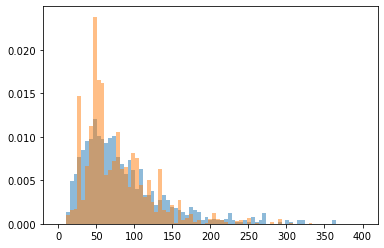Still a little off, although this might be due to bad sampling. Let's see how many of the decorrelated trajectories have this kind of transition.

In :
full_fixed_tree = ops_vis.PathTree(
fixed.steps,
ops_vis.ReplicaEvolution(replica=0)
)
full_history = full_fixed_tree.generator

In :
# start with the decorrelated tragectories
fixed_decorrelated = full_history.decorrelated_trajectories
# find the A->B transitions from the decorrelated trajectories
decorrelated_transitions = sum([flex_ens.split(traj) for traj in fixed_decorrelated], [])
# find the A->B transition from these which are decreasing
decorrelated_decreasing = sum([decreasing.split(traj) for traj in decorrelated_transitions], [])
print(len(decorrelated_decreasing))

114


So this is based off of 11 decorrelated trajectory transitions. That's not a lot of statistics.

However, we expect to see a very different distribution for the "increasing" paths:

In :
plt.hist([len(traj) for traj in categorized[increasing]], bins, density=True, alpha=0.5, color='g');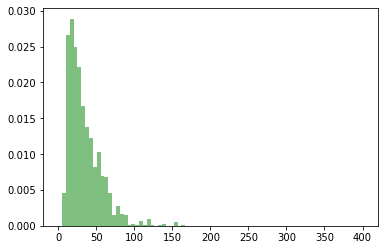Let's also check whether we go back and forth between the increasing transition and the decreasing transition, or whether there's just a single change from one type to the other.

In :
def find_switches(ensembles, trajectories):
switches = []
last_category = None
traj_num = 0
for traj in trajectories:
category = None
for ens in ensembles:
if len(ens.split(traj)) > 0:
if category is not None:
category = 'multiple'
else:
category = ens
if last_category != category:
switches.append((category, traj_num))
traj_num += 1
last_category = category
return switches

In :
switches = find_switches([increasing, decreasing], fixed_transition_segments)

In :
print([switch for switch in switches], len(fixed_transition_segments))

[0, 2, 39, 78, 394, 513, 584, 636, 711, 718, 1359, 1392, 1504, 1623, 1952, 2225, 2339, 2438, 2633, 2639, 2642, 2705, 2925, 2977, 3160, 3295, 3296, 3371, 3723, 3812, 4094, 4208, 4942, 4955, 5005, 5232, 5245, 5349, 5511, 5520, 5576, 5655, 5697, 5745, 5839, 5848, 5931, 5958, 6000, 6012, 6214, 6244, 6485, 6503, 6538, 6545, 6629, 6635, 6905, 7338, 7756, 7775, 7967, 7974, 8008, 8049, 8065, 8296, 8524, 8530, 8539, 8591, 9051, 9252, 9287, 9293, 9343, 9349, 9634, 9637, 9912, 10088] 10232


So there are a lot of switches early in the simulation, and then it gets stuck in one state for much longer.

Even though we know the alanine dipeptide transitions are not particularly rare, this does give us reason to re-check the temperature. First we'll check what the intergrator says its temperature is, then we'll calculate the temperature based on the kinetic energy of every 50th trajectory.

Note that the code below is specific to using the OpenMM engine.

In :
every_50th_trajectory = [step.active.trajectory for step in fixed.steps[::50]]

In :
# make a set to remove duplicates, if trajs aren't decorrelated
every_50th_traj_snapshots = list(set(sum(every_50th_trajectory, [])))
# sadly, it looks like that trick with set doesn't do any good here

In :
temperatures = [snap.instantaneous_temperature for snap in tqdm(every_50th_traj_snapshots)]


In :
plt.plot([T / T.unit for T in temperatures])
mean_T = np.mean(temperatures)
plt.plot([mean_T / mean_T.unit]*len(temperatures), 'r')
print("Mean temperature:", np.mean(temperatures).format("%.2f"))

Mean temperature: 300.40 K